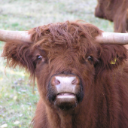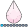JacobSimonsen
Barite | Level 11

## how I change the title in proc template to show a label of a variable instead of the variable name

Hello everyone,

Im trying to make a small change to the template of a statistical graphic plot. In the IIC plot from proc IRT I want the titles of each plot to be the variables labels instead of the variable name.

I found the template that produce the plot, and also the statement that produce the title.

In the code below, _ITEM0 will write the variable name in the title. How can I change that to the label of that variable?

``````
proc template;
source Stat.irt.Graphics.iteminfocurvepanel;
define statgraph Stat.IRT.Graphics.iteminfocurvepanel;
notes "Plots of Item Curves ";
dynamic _nItem _nRow _nCol _item0 _item1 _item2 _item3 _item4 _item5 _xmin _xmax _ymax _height _byline_ _bytitle_ _byfootnote_
_itemlabel1;
BeginGraph / designheight=_HEIGHT;
…
…
…
...
if (_NITEM>0)
cell;
entry halign=center  _ITEM0 / textattrs=GRAPHLABELTEXT;
Layout overlay / yaxisopts=(display=none) xaxisopts=(display=(line ticks tickvalues));
seriesplot x=TRAIT y=ITEMINFOI0;
endlayout;
endcell;
endif;
..
...
...
...
run;
``````
5 REPLIES 5

## Re: how I change the title in proc template to show a label of a variable instead of the variable na

Well, its not really to do with the template, as that just takes in a dynamic value.  Its more about you sgrender call.  So you could:

http://support.sas.com/documentation/cdl/en/lrdict/64316/HTML/default/viewer.htm#a000127812.htm

Call label in a datastep before into a macro variable and do it that way:

```data _null_;
set have (obs=1);
call symput('lb',call label(yourvar));
run;

proc sgrender...
define _item0="&lb."...
run;```

(Note not tested!!).  Although I would really say that if you are using a label in title (or anywhere, then its probably better to put that as string data in your data, then calling the sgrender with a variable - this way its flexible to use whatever is in the variable, and you don't need macro.JacobSimonsen
Barite | Level 11

## Re: how I change the title in proc template to show a label of a variable instead of the variable na

Thank you. Yes, I think you are right. That the sgrender procedure is called by the IRT procedure, not by me directly.

Further, IRT doesnt output the dataset used for the iteminformation curves, so I can not even call the sgrender procedure myself.JacobSimonsen
Barite | Level 11

## Re: how I change the title in proc template to show a label of a variable instead of the variable na

OK, I think I understood now. The problem was something different than what I original asked for.

The iteminformation curves can be shown by proc irt. Proc IRT produce a dataset containing the values, and it then call proc sgrender with this dataset, and some dynamic value.

The dataset, which I actually can get out with a "ods output" statement, is very bad organized. It has only four variables, which then contains information for all items I have. So, if I have lets say 8 items, then the first 101 observations contains information values for the first 4 items, and the next 101 observations contains information for the last 4 items. And, no labels.

It now need some cumbersome, but simple work to get a nice graph out of this.

## Re: how I change the title in proc template to show a label of a variable instead of the variable na

Sorry, never used proc irt, so do not know what the output from it is.  I would suggest starting by posting some example test data in the form of a datastep and show what you want out from it, so at least there is something to wor with.JacobSimonsen
Barite | Level 11

## Re: how I change the title in proc template to show a label of a variable instead of the variable na

Im not able show share the real data. But with simulated data I can show you here what I mean:

``````*simulate a dataset;
data simulation;
array q{8,4} _temporary_;
array alpha{4} _temporary_;

array item{8} 3;
array probability{5} _temporary_;
label item1="Anxiety" item2="Depression" item3="Hostility" item4="Guilt feelings" item5="Hallucinations" item6="Emotional withdrawal" item7="Grandiosity" item8="Disorientation";
do i=1 to dim(q);
do k=1 to dim(alpha);
alpha[k]=rand('normal',0,1);
end;
call sortn(of alpha(*));
do k=1 to dim(alpha);
q[i,k]=alpha[k];
end;
end;

do person=1 to 200;
p=rand('normal',0,1);
do i=1 to dim(item);
l=ifn(i=1,0.25,1);
do k=1 to 5;
if k=1 then probability[k]=cdf('logistic',alpha,l*p,1);
else if k<5 then  probability[k]=cdf('logistic',alpha[k],l*p,1)-cdf('logistic',alpha[k-1],l*p,1);
else if k=5 then probability[k]=1-cdf('logistic',alpha[k-1],l*p,1);
end;
item[i]=rand('table',probability,probability,probability,probability,probability);
end;
output simulation;
end;
keep item:;
run;

ods output iteminfocurve=iteminfocurve;
var item:;
run;
*the iic curve is missing the labels.

*Using the iteminfocurve dataset, we can put in the labels ;
proc format;
value item 1="Anxiety" 2="Depression" 3="Hostility" 4="Guilt feelings" 5="Hallucinations" 6="Emotional withdrawal" 7="Grandiosity" 8="Disorientation";
run;
data iteminfocurve2;
set iteminfocurve(in=a1 obs=101 keep=trait ItemInfoI0 rename=(ItemInfoI0=iteminfo) )
iteminfocurve(in=a2 obs=101 keep=trait ItemInfoI1 rename=(ItemInfoI1=iteminfo))
iteminfocurve(in=a3 obs=101 keep=trait ItemInfoI2 rename=(ItemInfoI2=iteminfo))
iteminfocurve(in=a4 obs=101 keep=trait ItemInfoI3 rename=(ItemInfoI3=iteminfo))
iteminfocurve(in=a5 firstobs=102 keep=trait ItemInfoI0 rename=(ItemInfoI0=iteminfo))
iteminfocurve(in=a6 firstobs=102 keep=trait ItemInfoI1 rename=(ItemInfoI1=iteminfo))
iteminfocurve(in=a7 firstobs=102 keep=trait ItemInfoI2 rename=(ItemInfoI2=iteminfo))
iteminfocurve(in=a8 firstobs=102 keep=trait ItemInfoI3 rename=(ItemInfoI3=iteminfo));
format item item.;
item=1*a1+2*a2+3*a3+4*a4+5*a5+6*a6+7*a7+8*a8;
run;
proc sgpanel data=iteminfocurve2;
panelby item;
series x=trait y=iteminfo;
run;``````
Discussion stats
• 5 replies
• 912 views
• 1 like
• 2 in conversation